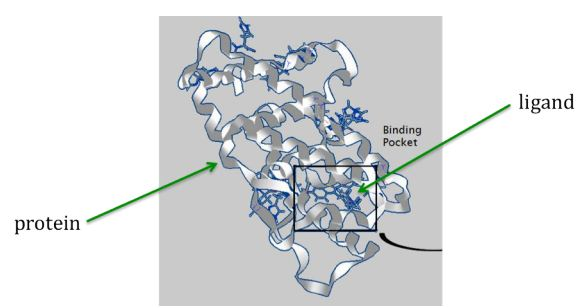# CHAPTER 6: Performance Study - Drug Design Exemplar¶

Let’s look at a larger example. An important problem in Biology is that of drug design. The goal is to find small molecules, called ligands, that are good candidates for use as drugs.

This is a very rough simulation of a program to compute how well a set of short protein ligands (each a possible drug) matches a given longer protein string. In the real software programs that do this, the matching is quite sophisticated, targeting possible ‘receptor’ sites on the protein.

Here is an image illustrating the concept of the ligand (represented by small sticks in center) binding to areas of the protein (represented by ribbon structure):For the real versions of this code and our simulated case, the longer the ligand or the longer the protein, the longer it takes for the matching and score of the match to complete.

We have created a default fake protein in the code. This can be changed on the command line.

We create the list of possible ligands by choosing random lengths (controlled by the maxligand variable). Thus, each ligand can be of a different length.

## 6.1 The Drug Design Exemplar¶

There is a folder in the RaspberryPiBasics directory called drugdesign. To get to this directory from the integration directory, use the command

cd ../drugdesign


Typing ls in this directory should reveal three additional directories:

• drugdesign-dynamic: an OpenMP implementation using dynamic scheduling of ligands

• drugdesign-static: an OpenMP implementation using static scheduling of ligands

• drugdesign-threads, an implementation using C++11 threads (std::thread).

Each directory contains a Makefile to build the code along with the raw source code.

Unlike previous code examples, the drug design exemplar is implemented in C++, not C. Second, our primary focus this chapter is analyzing the performance of the static and dynamic OpenMP programs.

## 6.2 Speedup¶

Speedup is the ratio of the execution time of a serial program to its parallel execution. Specifically,

$S_n = \frac{T_1}{T_n}$

Where $$T_1$$ is the time it takes to execute a program on 1 thread, while $$T_n$$ is the time it takes to execute a program on n threads. Some important notes:

• A speedup greater than 1 indicates that there is benefit to the parallel implementation. A speedup of x means that the parallel code is x times faster.

• A speedup less than 1 indicates that there is no benefit to parallelism.

To correctly calculate speedup, we must first measure the running time of a program. In this case, we will use the time -p command. The Linux time command prints out system resource usage for programs. In this case, we are interested in the wall clock time (real), which reflects the total amount of time that the program ran.

For example, to run the the drugdesign-static executable on 1 thread, cd into the drugdesign-static directory, type make, and then run the following command:

time -p ./drugdesign-static 1 6


The second parameter indicates what the maxligand size should be. Please use the number 6 as the second parameter throughout all your benchmarks.

## 6.3 Performance Study¶

Exercise 1:

Fill out the table (on a separate piece of paper) by running the following series of tests:

Time (s)

drugdesign-static

drugdesign-dynamic

Exercise 2:

Exercise 3:

We will use Python to assist us with our speedup calculation. Fill in the array values in code below to compute the speedup for each version on each set of threads:

## 6.4 Summary - Static vs. Dynamic Scheduling¶

In many cases, static scheduling is sufficient. However, there is an implicit assumption with static scheduling that all components take about the same amount of time. However, if some components take longer than others, a load balancing issue can arise. In the case of the drug design example, different ligands take longer to compute than others. Therefore, a dynamic scheduling approach is better.

Next, note that speedup is non-linear, especially at higher number of threads. While increasing the number of threads is supposed to generally improve the run time of a program, it usually does not scale linearly with the number of cores. This occurs for a number of reasons. First, at higher number of threads, it is more likely that the serial components of a program (such as thread creation overhead, and any other necessary serial operations that must take place before the parallel code can run) start dominating run time (see Amdahl’s Law). Second, resource contention on the CPU or memory from other processes can cause slow downs. A related measure called efficiency measures how well a program uses the cores assigned to it.

Further Reading on Performance: Dive into Systems, Chapter 14.4

You have attempted of activities on this page